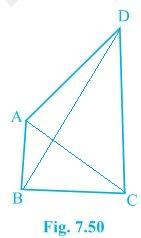# AB and CD are respectively the smallest and longest sides of a quadrilateral ABCD (see Fig.) . Show that A > C and B > D.#### Solution

Given

From ΔABD,

So, ADB < ABD — (i) (Since angle opposite to longer side is always larger)

From ΔBCD,

BC < DC < BD

Hence, it can be inferred that

BDC < CBD — (ii)

On adding equation (i) and equation (ii) we get,

ADB + BDC < ABD + CBD

B > D

From Δ ABC,

ACB < BAC — (iii) (Since the angle opposite to the longer side is always larger)

DCA < DAC — (iv)

On adding equation (iii) and equation (iv) we get,

ACB + DCA < BAC+DAC

∴ A > C

Hence Proved